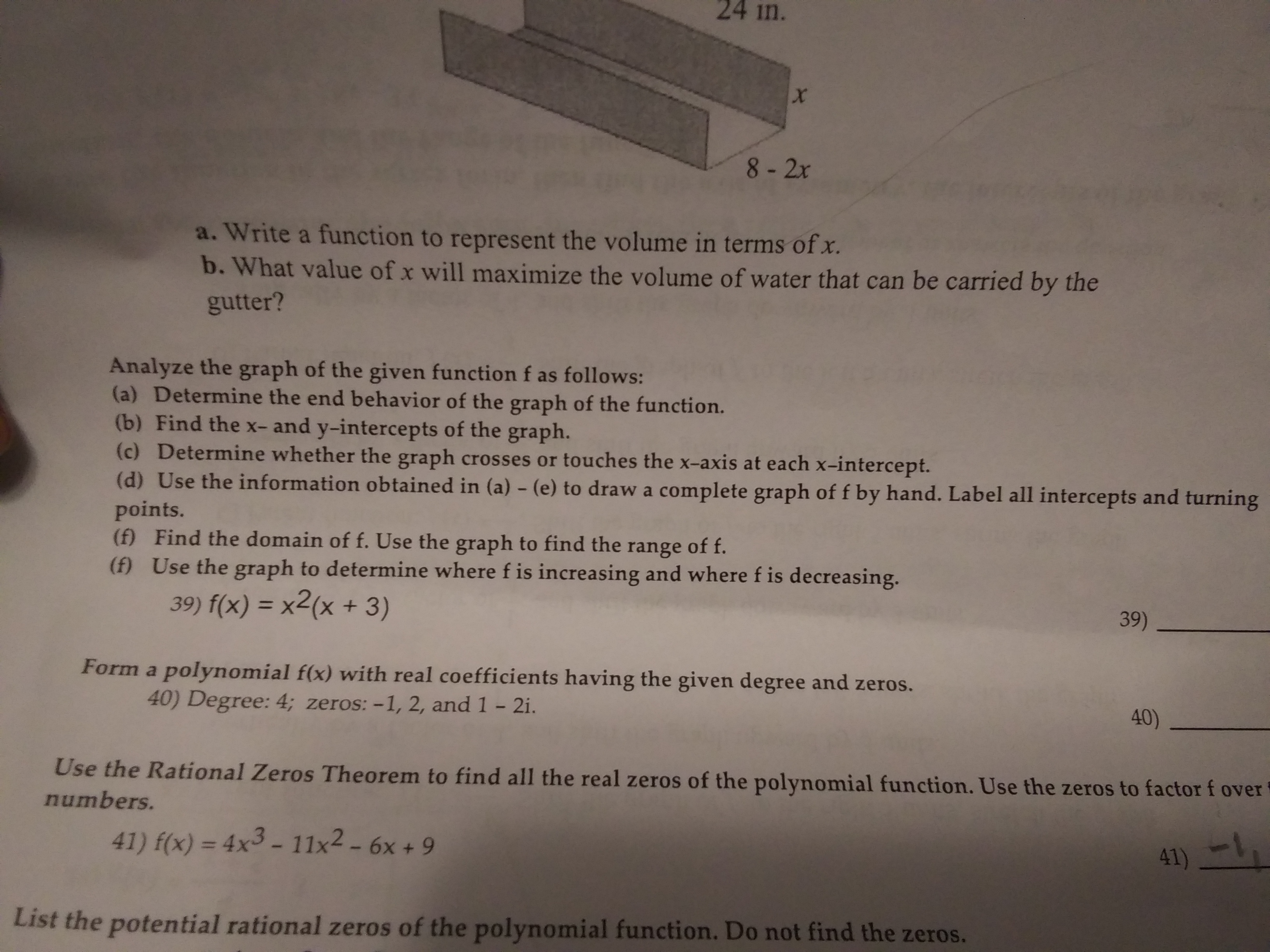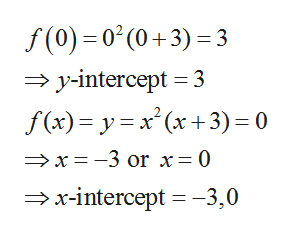# 24 in.8- 2xa. Write a function to represent the volume in terms of x.b. What value of x will maximize the volume of water that can be carried by thegutter?Analyze the graph of the given function f as follows:(a) Determine the end behavior of the graph of the function.(b) Find the x- and y-intercepts of the graph.(c) Determine whether the graph crosses or touches the x-axis at each x-intercept.(d) Use the information obtained in (a) - (e) to draw a complete graph of f by hand. Label all intercepts and turningpoints.(f) Find the domain of f. Use the graph to find the(f) Use the graph to determine where f is increasing and where f is decreasing.range of f.39) f(x) = x2(x + 3)%3D39)Form a polynomial f(x) with real coefficients having the given degree and zeros.40) Degree: 4; zeros: -1, 2, and 1 - 2i.40)Use the Rational Zeros Theorem to find all the real zeros of the polynomial function. Use the zeros to factor f overnumbers.41) f(x) = 4x3 - 11x2 – 6x + 941)List the potential rational zeros of the polynomial function. Do not find the zeros.

Question
15 views

F(x)=x2(x+3)

1. Determine the end behavior of the graph of the function
2. Find the x- and y- intercepts of the graph
3. Determine whether the graph crosses or touches the x-axis at each x-intercept.
4. Use the information obtained in (a)-(e) to draw a complete graoh of f by hand. Label all intercepts and turning points
5. Find the domain of f. Use the graph to find the range of f.
6. Use the graph to determine where f is increasing and where f is decreasing.help_outlineImage Transcriptionclose24 in. 8- 2x a. Write a function to represent the volume in terms of x. b. What value of x will maximize the volume of water that can be carried by the gutter? Analyze the graph of the given function f as follows: (a) Determine the end behavior of the graph of the function. (b) Find the x- and y-intercepts of the graph. (c) Determine whether the graph crosses or touches the x-axis at each x-intercept. (d) Use the information obtained in (a) - (e) to draw a complete graph of f by hand. Label all intercepts and turning points. (f) Find the domain of f. Use the graph to find the (f) Use the graph to determine where f is increasing and where f is decreasing. range of f. 39) f(x) = x2(x + 3) %3D 39) Form a polynomial f(x) with real coefficients having the given degree and zeros. 40) Degree: 4; zeros: -1, 2, and 1 - 2i. 40) Use the Rational Zeros Theorem to find all the real zeros of the polynomial function. Use the zeros to factor f over numbers. 41) f(x) = 4x3 - 11x2 – 6x + 9 41) List the potential rational zeros of the polynomial function. Do not find the zeros. fullscreen
check_circle

Step 1

The given function is

Step 2

(a)

Observe that for large positive values of variable x, the value of the function is a large positive number and further increase in the value of the variable will only increase value of the function. Thus the right end behaviour of the function is that it tends to infinity as input x tends to infinity.

Similarly observe that for large negative values of variable x, the value of the function is a large negative number and further decrease in the value of the variable will only decrease value of the function. Thus the left end behaviour of the function is that it tends to negative infinity as input x tends to negative infinity.

Step 3

A y-intercept can be found by finding f(0) and an x-int...help_outlineImage Transcriptionclosef(0) = 0° (0 +3) = 3 → y-intercept = 3 f (x)= y= x' (x +3)= 0 →x= -3 or x=0 →x-intercept = -3,0 fullscreen

### Want to see the full answer?

See Solution

#### Want to see this answer and more?

Solutions are written by subject experts who are available 24/7. Questions are typically answered within 1 hour.*

See Solution
*Response times may vary by subject and question.
Tagged in

### Algebra